# Interior Angles of Polygons

A polygon is any plane (flat) shape with straight edges. The interior angles of polygons are the angles on the inside of the polygon, between any pair of edges that meet at a point. Here are some examples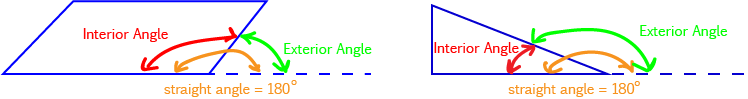In the above pictures, the dotted line is the extension of an edge of the polygon. You might notice that there are two other angles marked: the exterior angles of the polygon are the angles outside the polygon between one edge and the extension of the next. A straight angle ($180^\circ$) is also marked. This is because the interior and exterior angles are angles along the same straight line, and so they add up to $180^\circ$. We call them supplementary angles.

## Triangles

The interior angles of a triangle always add up to $180^\circ$

We call the sum of the interior angles of a polygon its angle sum, so we could also say

The angle sum of a triangle is $180^\circ$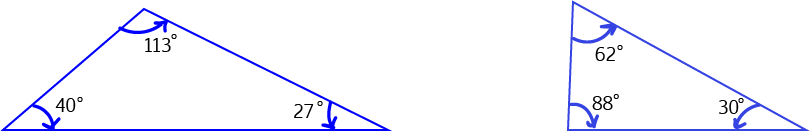In the pictures, the sum of the interior angles of the triangle on the left is $40^\circ + 113^\circ + 27^\circ = 180^\circ$.>

The sum of the interior angles of the triangle on the right is also $62^\circ + 88^\circ + 30^\circ = 180^\circ$.

A quadrilateral is a 4-sided polygon. Some examples of quadrilaterals are squares, rectangles, parallelograms and trapeziums.

The interior angles of a quadrilateral always add up to $360^\circ$

We call the sum of the interior angles of a polygon its angle sum, so we could also say

The angle sum of a quadrilateral is $360^\circ$

We can see this by dividing the quadrilateral up into two triangles so that the corners of the triangles occur at the corners of the quadrilateral: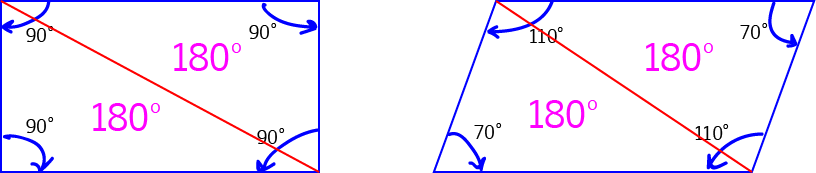As the angle sum of each triangle is $180^\circ$, the angle sum of the quadrilateral is equal to $2 \times 180^\circ = 360^\circ$.

Here are some examples of the angles in quadrilaterals. I've drawn a rectangle, and then tilted it by $20^\circ$ to form a parallelogram: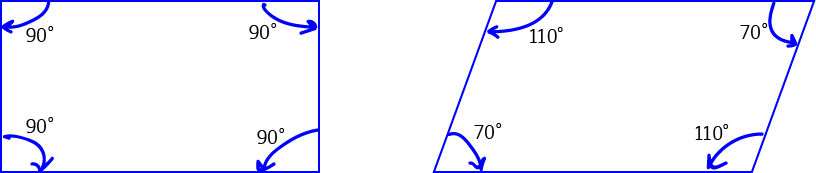In the pictures, the sum of the interior angles of the quadrilateral (rectangle) on the left is $90^\circ + 90^\circ + 90^\circ + 90^\circ = 360^\circ$.>

The sum of the interior angles of the quadrilateral (parallelogram) on the right is also $70^\circ + 110^\circ + 70^\circ + 110^\circ = 360^\circ$. Can you see how two of the angles have been made smaller by $20^\circ$ and two have been made larger by $20^\circ$ because of the tilting? The angle sum remains the same.

## Angle Sums of Polygons With More Edges

You can do the same thing with polygons with more edges. Divide them up into triangles so that the vertices of the triangles coincide with the vertices of the polygon, count the number of triangles and then multiply it by $180^\circ$ (the angle sum of a triangle) to give the angle sum of the polygon.

The picture shows the results for a pentagon and a hexagon: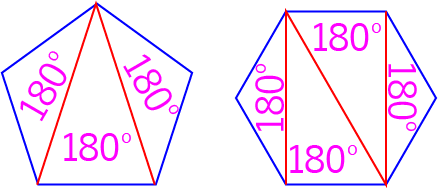We split the pentagon up into 3 triangles, so the angle sum of a pentagon is $3 \times 180^\circ = 540^\circ$.

The hexagon was split up into 4 triangles, so its angle sum is $4 \times 180^\circ = 720^\circ$.

Are you starting to see a pattern here? What do you think the angle sum of a nonagon (nine-sided polygon) would be? You can check your answer in the table that appears next.

## Interior Angles of Regular Polygons

A regular polygon is a polygon in which every side has the same length, and every interior angle has the same size. We can use the angle sums of polygons to work out the sizes of the interior angles of regular polygons.

For example, the angle sum of a pentagon is $540^\circ$, so every interior angle of a regular pentagon will be equal to $540 \div 5 = 108^\circ$.

The angle sum of a hexagon is $720^\circ$, so every interior angle of a regular hexagon will be $720 \div 6 = 120^\circ$.

## The General Rule for Angle Sum of a Polygon

Each time we increase the number of sides of the polygon by 1, we also increase the number of triangles that we can split it up into by 1. This means that we add $180^\circ$ to the angle sum of the polygon. If the polygon is regular, then all interior angles are equal, and we can work out the size of each interior angle by dividing the angle sum by the number of sides of the polygon (which is equal to the number of interior angles).

The table below shows the angle sums of polygons of various sizes, and the sizes of the interior angles of various polygons:

Name of Polygon Number of Sides Angle Sum Picture Interior Angles if Regular
Triangle 3 $180^\circ$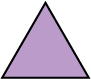$60^\circ$
Quadrilateral 4 $360^\circ$$90^\circ$
Pentagon 5 $540^\circ$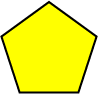$108^\circ$
Hexagon 6 $720^\circ$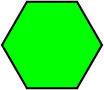$120^\circ$
Heptagon (or Septagon) 7 $900^\circ$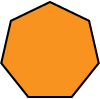$128.57\dots^\circ$
Octagon 8 $1080^\circ$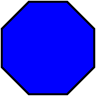$135^\circ$
Nonagon 9 $1260^\circ$$140^\circ$
Decagon 10 $1440^\circ$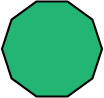$144^\circ$

In general, we can divide a polygon with $n$ sides up into $n - 2$ triangles, so

\begin{align*} \text{Angle Sum} &= (n - 2) \times 180^\circ\\ \text{Angles of Regular } n\text{-gon} &= \dfrac{(n - 2) \times 180^\circ}{n} \end{align*}

### Example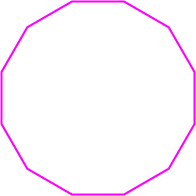Find the angle sum and size of each interior angle of a regular dodecagon.

Solution:

A dodecagon has 12 sides, so its angle sum is $(12 - 2) \times 180 = 1,800^\circ$.

The size of each interior angle of a regular dodecagon is given by $((12 - 2) \times 180)\div 12 = 150^\circ$.

### Description

In these chapters you will learn about plane geometry topics such as

• Area (Irregular polygons, plane shapes etc)
• Perimeter
• Conic sections (Circle, Ellipse, Hyperbola etc)
• Polygons (Congruent, polygons, similar, triangles etc)
• Transformations and symmetry (Reflection, symmetry, transformations etc)

etc

Even though these chapters are marked for Year 10 or higher students, several topics are for students in Year 8 or higher

### Audience

Year 10 or higher, suitable for Year 8 + students as well.

### Learning Objectives

You must be logged in as Student to ask a Question.